# Multicomputational Irreducibility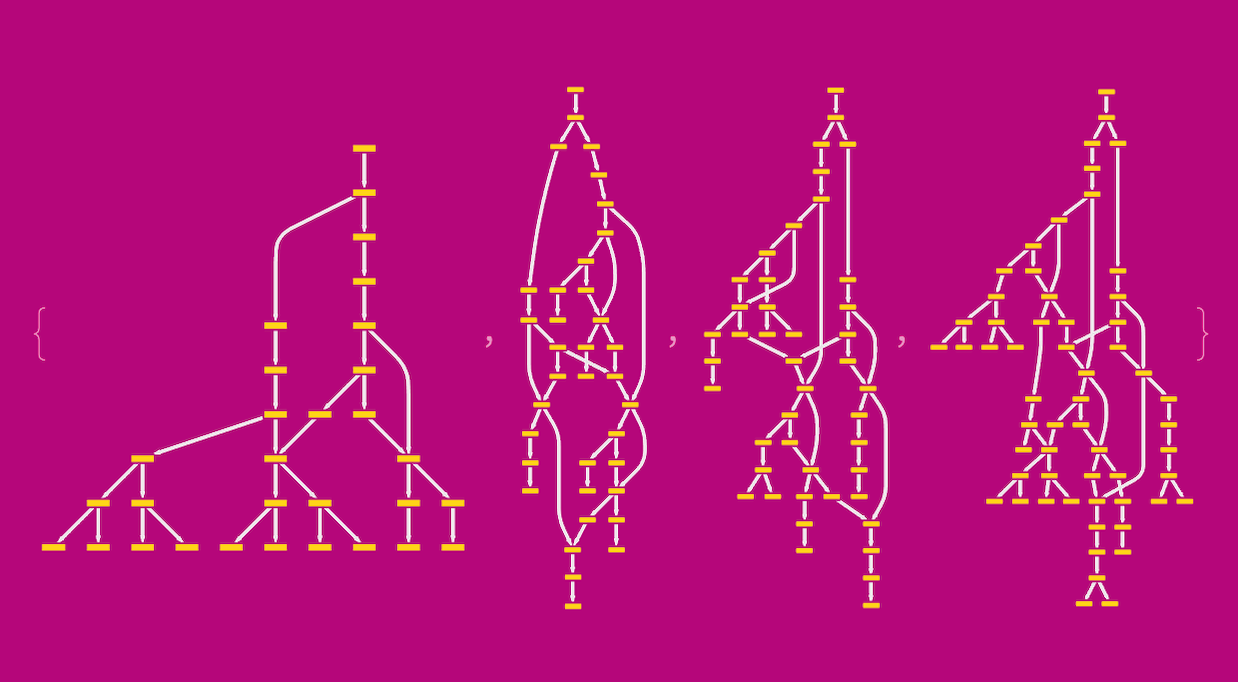Multicomputation is the cornerstone of much of the basic science that my teammates and I are doing with Stephen Wolfram. We see an opportunity to metamodel many areas of applied science using the multicomputational paradigm. In fact, the range of opportunities that we envisage is so wide that we are launching the Wolfram Institute in order to expand the effort beyond Wolfram Research. It’s a momentous period. But because multicomputation is still new, I feel a responsibility to help communicate in greater detail the aspects of multicomputation that I personally find to be compelling. And I have great reference material for doing so, because introducing a new paradigm of science is precisely what Stephen began to do in the 1980s with the computational paradigm. And I still refer to those works from the 1980s today because they cover then-novel concepts that have come to serve as guiding principles for the work that we do now. And a key concept, which distinguishes those papers from other theoretical literature on the study of computability, is that of computational irreducibility. So, now that we are developing a new paradigm that builds upon the one that Stephen pioneered decades ago, it seems appropriate to consider irreducibility in the multicomputational context.

## A Review of Computational Irreducibility

Computational irreducibility is the property of computational processes whose behaviors resist prediction. Although popularized through A New Kind of Science, the property was previously subject to considerable study in the 1980s, with the results published in several papers. Such results were surprising because they contradicted algorithmic information theory (AIT), which was in turn influenced by earlier works in information theory and computability theory. The key presumption of AIT, which Stephen’s findings contradicted, was that one could assign a value to the complexity of an algorithm by measuring its description length, where algorithms with shorter rules were presumed to produce outputs with greater regularity and less randomness. The finding that computations with simple rules could be autoplectic—that is, increasing in complexity without external influence—was surprising, and its implications extended beyond the theory of computation to the general study of physical systems. It cast doubt on a paradigmatic principle of modern science, namely that one, in the mathematical tradition, could design parsimonious models with few parameters that make the world tractable enough for humans to gain predictive power over it.

## Multicomputational Irreducibility

In what follows, we too will study the behavior of simple computational rules. However, our focus will not be placed on individual rules; rather, we will examine multicomputations that involve either multiple rules that are computed together or rules that admit multiple pattern matchings. In either case, multicomputations differ from their single-way predecessors in that they have parallel evaluation fronts. Let’s consider the case of a multicomputation that admits two different Turing machine (TM) rules: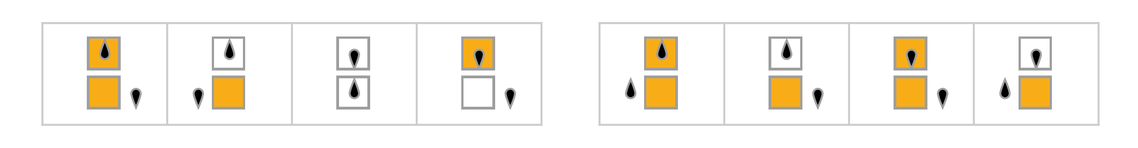✕

Let’s first run each TM rule separately for 25 steps: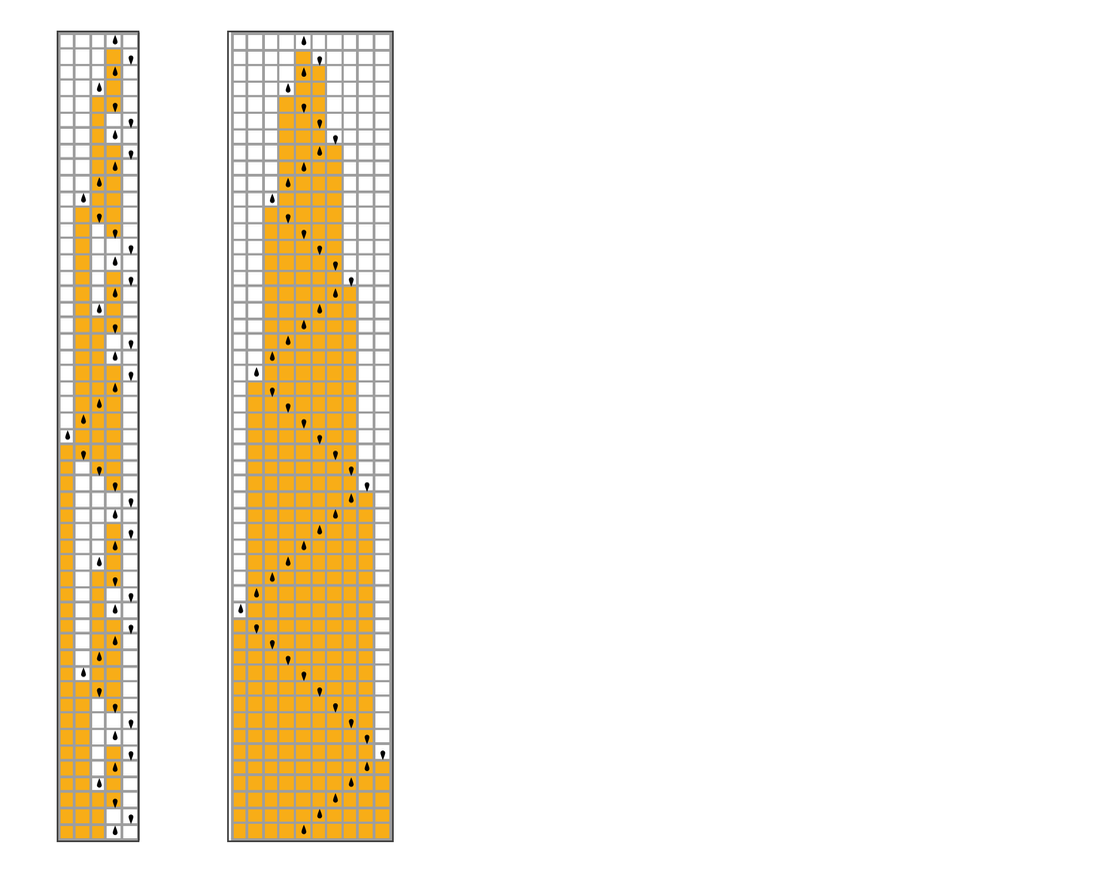✕

Looking at the individual TM plots, we see that the behavior shown in either case is predictable. Next, let’s contrast the behavior of the individual rules with the behavior of a multiway Turing machine (MTM) that accepts both rules, which we’ll run for 10 steps: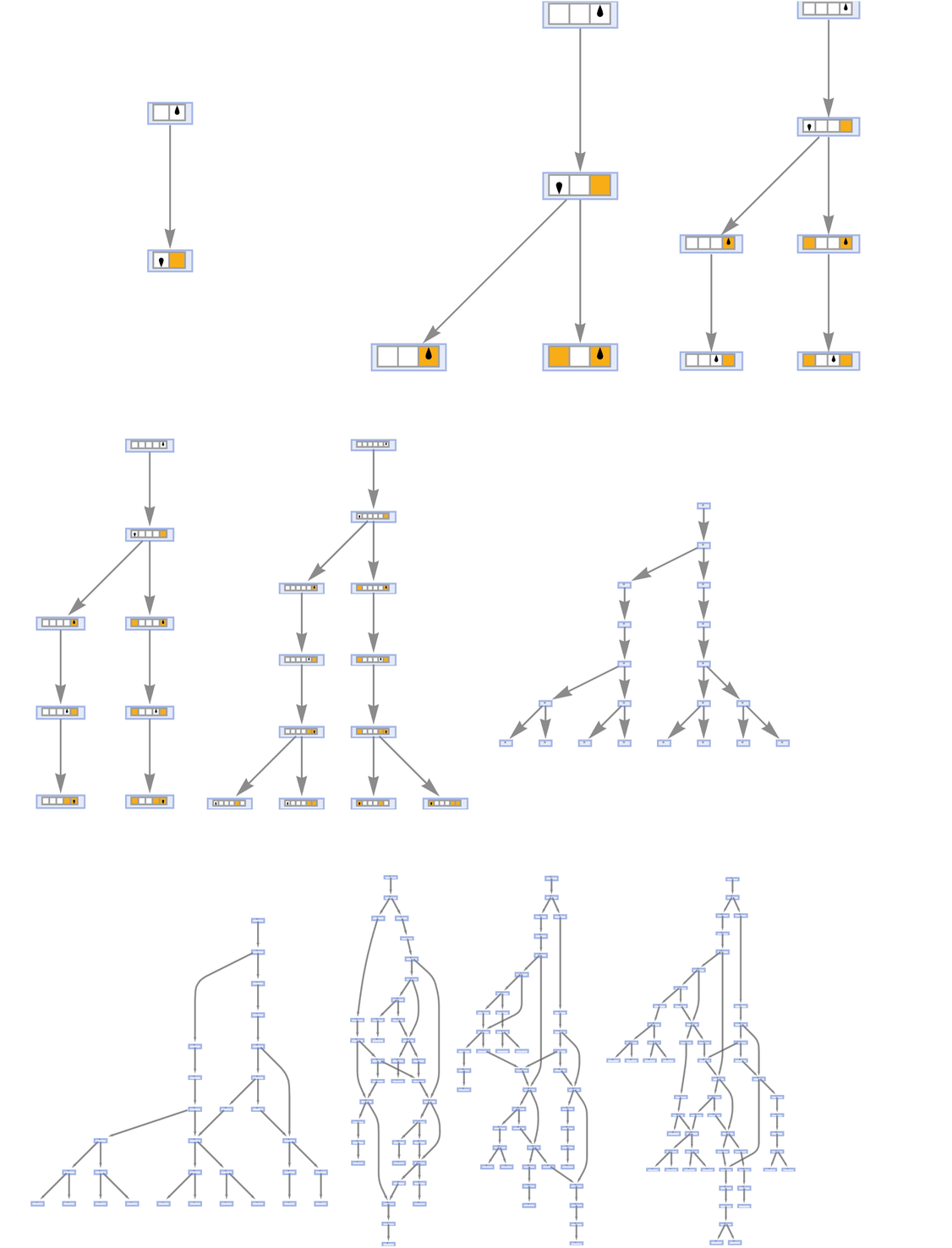✕

Intriguingly, the multicomputational behavior is more difficult to anticipate. We can predict how each rule behaves individually, but we cannot as readily predict the “interactions” between those rules. Thus, it appears as though multicomputations too can be either predictable or unpredictable, depending on the convergence and divergence of their parallel evaluation fronts. We will refer to the property of unpredictability for multicomputations as multicomputational irreducibility. Such a property might seem obvious, but it opens upon a trove of new questions about the behavioral study of computational rules (or, as we like to call it, ruliology) and its application to real-world systems (metamodeling).

## A Pure n-Machine Definition

Let us try to produce a slightly more formal definition of multicomputational irreducibility. In order to do so, we must think about an idealized machine, one that differs from those that we might usually encounter. The machines that we typically find in theoretical computer science are 1-machines. Graph-theoretically, we model their discrete computations as 0-spaces (vertices) and the overall program that they execute as a 1-space (a path). For a 1-machine, the initial condition is a vertex, as is the final state, and every state in between. A 2-machine, on the other hand, is a machine that computes over paths (1-spaces), with the overall computation forming a sheetlike 2-path (which, prima facie, is not unlike the terms of higher identity types constructed in univalent foundations).

Together, one can use 2-machines, 1-machines and higher n-machines to determine the path deformations, boundaries and geni of multicomputations, as one does in generalized homology with CW-complexes, homotopies and functors. And one can think of the “laps” performed by such machines over multicomputational graphs as playing a role similar to that of homotopy groups. But a key point here—as shall be explored later—is that such concepts are relevant to the experimental study of multicomputational irreducibility. For instance, a maximally confluent multicomputation consists of highly interdeformable paths; such a multicomputation is also easily predictable and thus multicomputationally reducible. As the multicomputation runs, we know that the paths are, and will continue to be, interdeformable.

On the other hand, with multicomputations for which 2-machine laps are non-Abelian (such that as the multicomputation proceeds, one cannot always “go back and forth” between paths), irreducibility is more likely. Because the paths are not all interdeformable, we do not know whether or not they will converge as the multicomputation continues to run. Another elementary example of reducible multicomputations is the class of fully ramified multicomputations that do not exhibit any confluence (and are thus entirely refractory to 2-machines); we can safely anticipate that confluence will never occur.

As of now, 2-machines and higher n-machines are idealizations. No known computational technologies can implement 2-machine capabilities. Nonetheless, 2-machines are useful for positing theoretical definitions. In particular, multicomputation can be defined with reference to such machines: a computation with multiple evaluation fronts is multicomputationally irreducible if its corresponding 2-machine computation is undecidable. Put informally, if as a multicomputation proceeds it is unclear if and how one could compute “horizontally” (pathwise) from one evaluation front to another, then the multicomputation is itself irreducible. But, on the other hand, if one always knows that all paths are interdeformable, then the corresponding 2-machine computation is decidable (positively so), as is the case if we know that the multicomputation is fully ramified and never converges (negatively so). Alternatively, as Stephen once put it during a conversation, a multicomputation is irreducible if, in order to know what paths a multicomputation gives, one must simply compute all paths.

However, we do have a way to study multicomputational irreducibility even without 2-machines: branchial space. By extracting from a multicomputation its branchial graph, we can examine the relations between paths, and are thus effectively performing a “2-machine branchial reduction,” or a conversion of an (infeasible) 2-machine computability problem into something that our Physics Project makes tractable. (And Jonathan Gorard’s branchial Turing machine offers a nice connection to the TM and MTM examples given previously.)

## Approximation of Multicomputational Irreducibility Using a Branchial Lyapunov Exponent

Branchial reductions of multicomputations provide a practical way to approximate irreducibility. In order to show how approximations as such can be done, let’s begin with some simple examples.

Consider the numerical multicomputation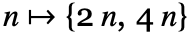. As we shall see, it is multicomputationally reducible, as its paths are all interdeformable. Here is the multicomputation, which we run for six steps:✕

And here are the branchial graphs generated at successive steps:✕

Evidently, the branchial graphs do not evolve whatsoever, and neither do their corresponding distance matrices (given as array plots here):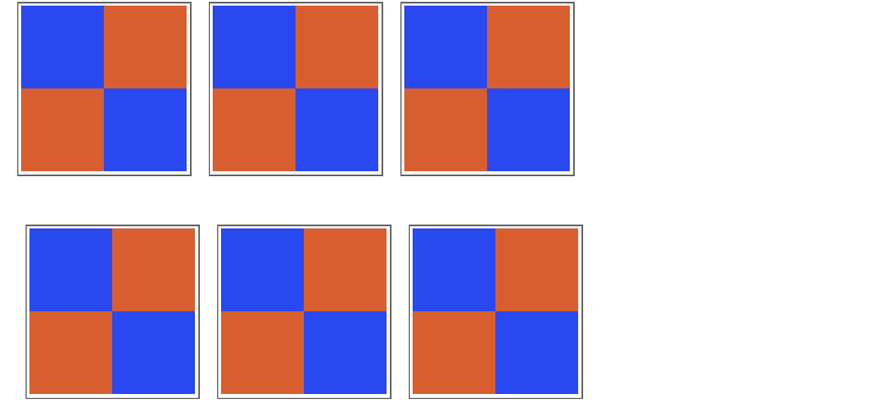✕

And we can predict (correctly) that such will continue to be the case, indefinitely, for all future steps. Graphs for which all paths are interdeformable are multicomputationally reducible, as discussed previously.

Next, consider a multicomputation that is entirely ramified: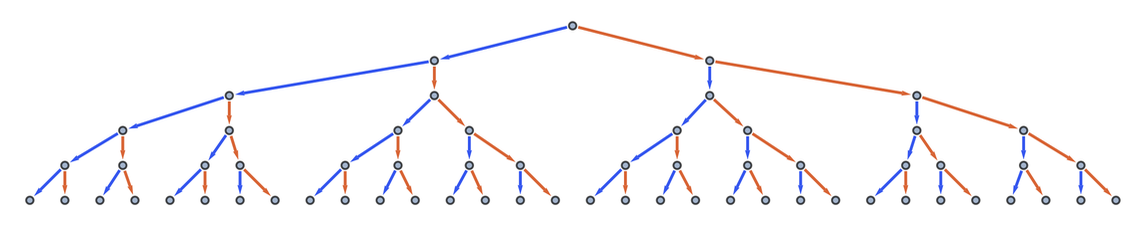✕

The corresponding branchial graphs consist of disconnected graph segments, each containing two vertices: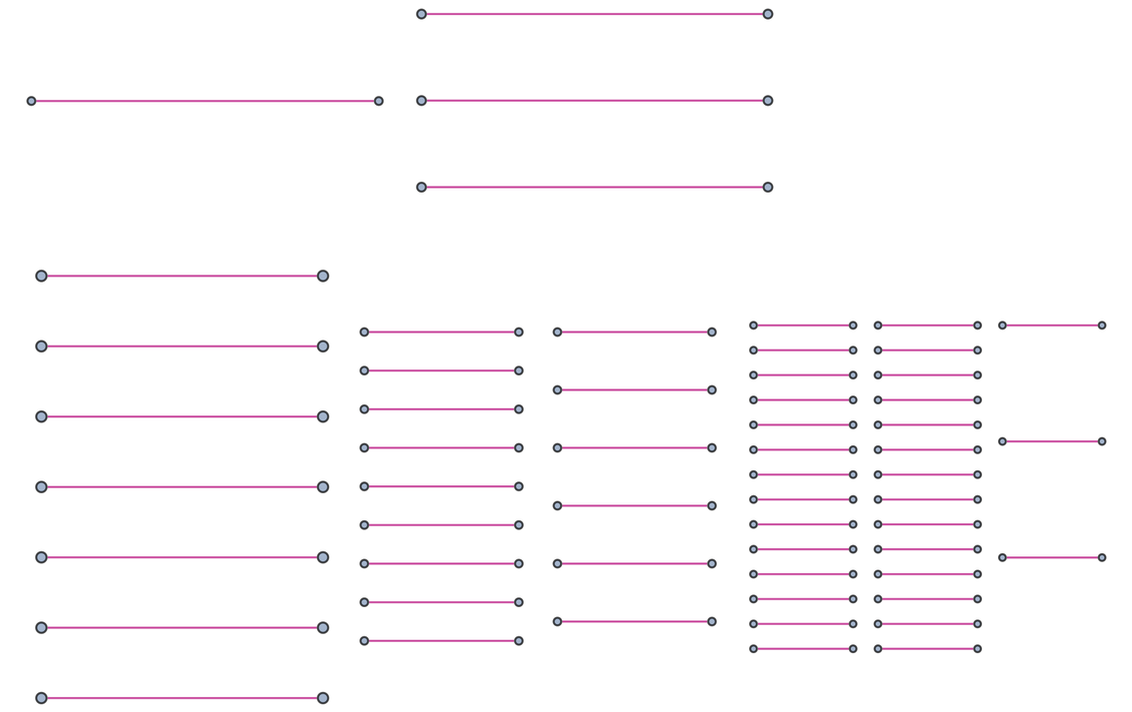✕

This behavior is also predictable. At step t, the number of segments in the branchial space is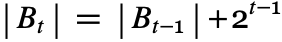, where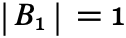. A general remark: if one can readily provide an equation that predicts future branchial behavior, it is clear that one has found a case of multicomputational reducibility.

Here’s another view of the same branchial evolution: an array plot of the graph distance matrices for each step. In this case, we see that the distance-1 branchial relation (i.e. the segment) is “propagated” consistently: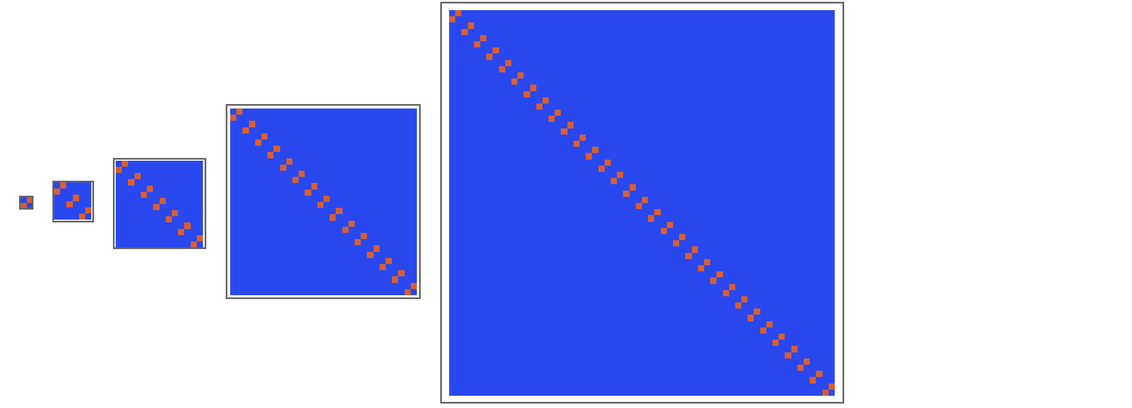✕

One initial test that Stephen performed when studying randomness and autoplectic behavior among cellular automata is, among other tests, the study of their Lyapunov exponents. In the case of elementary cellular automata, one can measure Lyapunov exponents by calculating the slopes of a computation. In order to study multicomputational irreducibility, we can introduce a branchial analog of the Lyapunov exponent, denoted by λB.

Lyapunov exponents measure the “drift” of initial conditions in dynamical systems. When we perform a branchial reduction of a 2-computation, the initial condition is the branchial graph at step 1, which in this case is a single branchial edge with two vertices (i.e. the segment). And, as we can see in this case, the initial distance does not “drift” as we iterate the ramified multicomputation given previously: the one-unit distance segment is simply “propagated” along the matrix diagonal. As a result, the array plot appears “linear” in that the same branchial distance is “passed” from one branchial pair to another. If, however, the branchial graph assumed a more elaborate, connected graphical configuration, then the branchial evolution would “drift” from the initial condition, with matrix entries appearing farther from the diagonal (as we’ll see shortly).

We can also describe λB more formally. Consider branchial graph distance matrix entries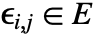for a distance matrix D = r · c. Now, consider only those entries for which the distance matrix value d is 1 (which constitute the entries of the adjacency matrix):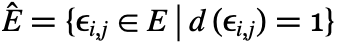Row and column positions of each entry are obtained via a position query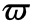: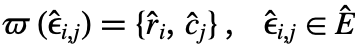And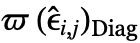yields the positions nearest to the diagonal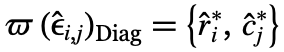where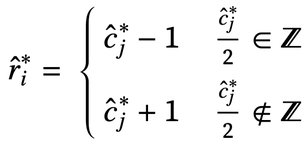&#10005

We measure λBi by taking, for each i: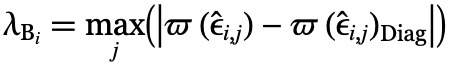In the case of our ramified multicomputation, for each node in the branchial graph, λB = 0: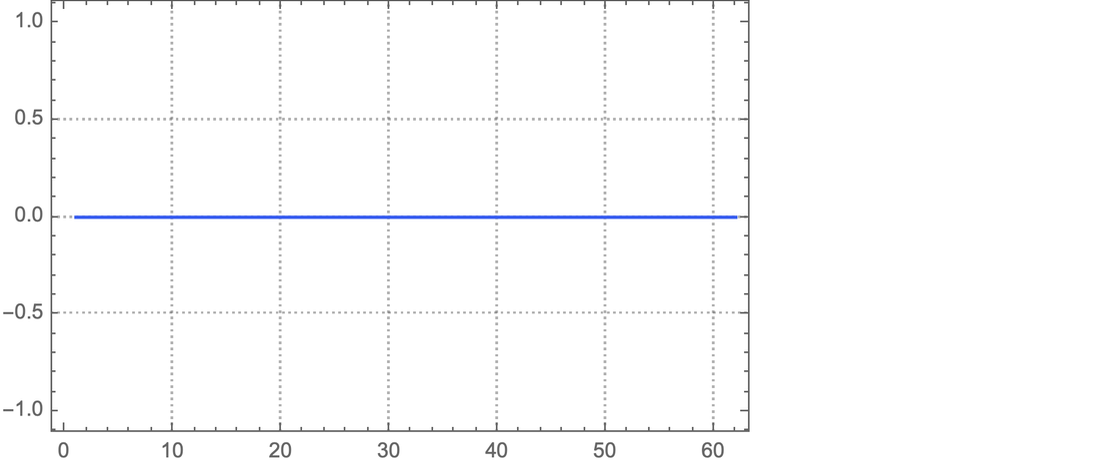✕

And in general, “λB plot flatness” is a visual heuristic for multicomputational reducibility. In the case of the ramified multicomputation, the result is not surprising. We can see that confluence will never happen; thus, the multicomputation is reducible.

Next, let us consider multicomputations with nontrivial confluence, such as this one, which we run for seven steps: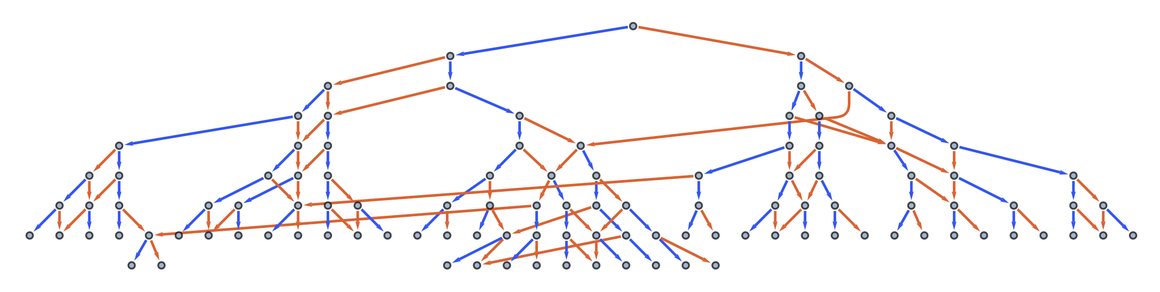✕

We see that the branchial distance matrices exhibit slightly more “complex” behavior and cannot be as easily predicted: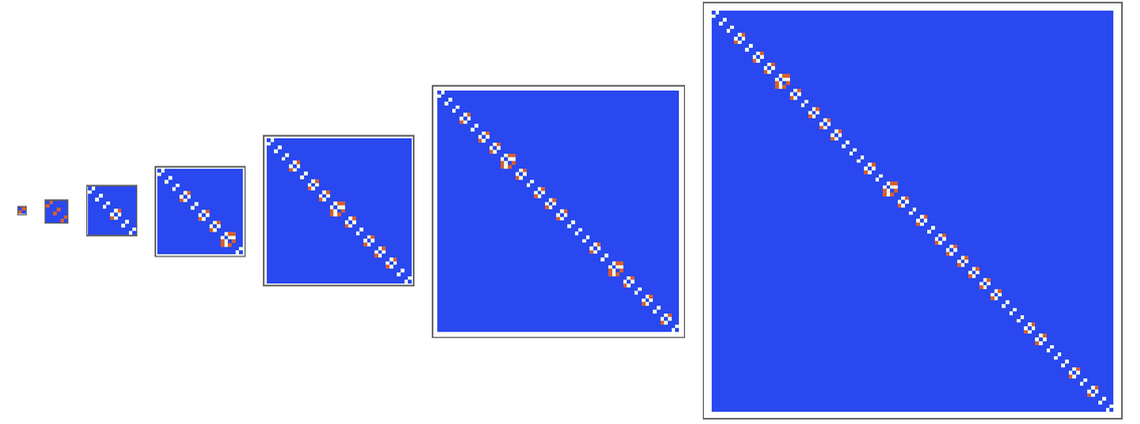✕

Consider now the following multicomputation: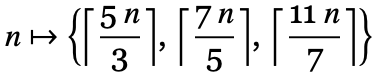Its branchial graphs are nontrivial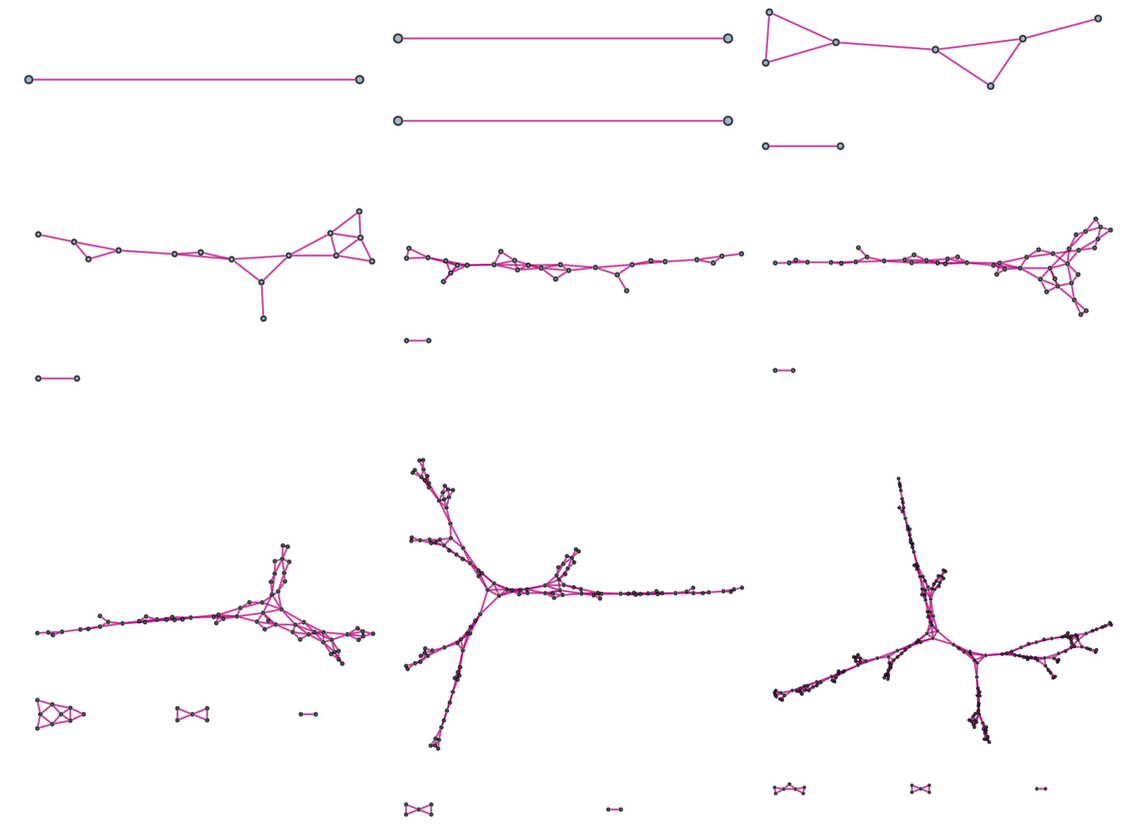✕

as are their corresponding distance matrices: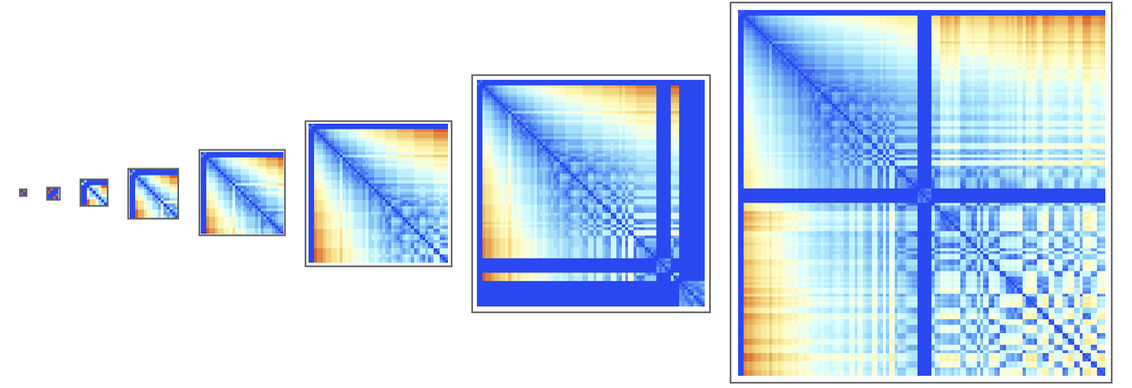✕

Notice here that the original condition, the branchial segment, is not simply “passed” to other segments. With time, as the branchial graph takes shape, there are many branchial vertices that are one branchial unit of distance away from others in a large connected component. Thus, the initial condition is effectively “diffused” throughout branchial space, rather than just being “propagated” at each step in segmentary form.

Here are the λBi values for the branchial graph obtained after 10 steps: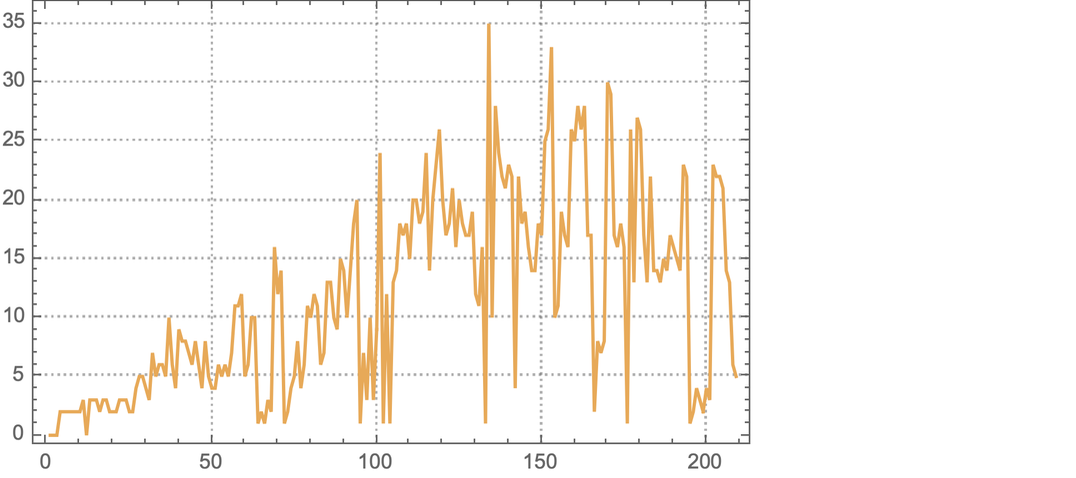✕

As one can see, this plot is far from being flat.

One should keep in mind that our distance matrices can be permuted, and that the distance matrices that we obtain by default in the Wolfram Language correspond to a default assignment of vertex numberings. But it so happens that the matrices that we obtain by default make it particularly easy to study the predictability of branchial behavior. Nik Murzin has suggested that an ensemble-like λB measure could be obtained by considering all possible matrix permutations. Such an approach will be subject to further study.

## Foliation as Multicomputational Choice

Multicomputational research differs from computational research in a number of ways. One key difference between the two is that we enjoy the prerogative of choice when doing multicomputational research; there are different options available to us that aren’t available when we study single-way computations. When one runs a single computation—and an irreducible one in particular—one has little choice other than to run it and study its behavior. But in the multicomputational case, one is able to make choices in how one studies behavior. This is the case because, when one computes multiple evaluation fronts, one can “synchronize” or “coordinate” multicomputational states in different ways.

And yes—we actually do have a choice in this matter. One might presume that, when we run multiple rules, it is simply the case that all states “from step 1” of each computation are synchronized, all states that result “from step 2” are synchronized and so on. But, as will be shown, one has a choice in the “simultaneity orientation” that dictates which vertices in the multicomputational graph “occur together” at each step.

The researcher makes such choices by selecting a foliation. Originally, the foliation was proposed as a theoretical concept and computational technique for the Physics Project. But we have since come to understand that foliation choices can be made for all multicomputations. Curiously, with multicomputation, we are making the study of computational behavior even more “complex” than before by building systems with many rules (or rules that can be evaluated in different ways); nevertheless, by doing so, we reintroduce a prerogative of choice into our computational methodology that we do not have when we study rules individually. And we anticipate that choice as such affords tremendous metamodeling advantages. For instance, we think that multicomputation makes it possible to capture the aggregate behavior of systems, so long as one selects the right foliation. And we believe that observation and the general transduction of all systems can be metamodeled this way.

But why is choice of foliation important? As we will see, λB approximations for the same multicomputation differ by foliation. For instance, let’s revisit the multicomputation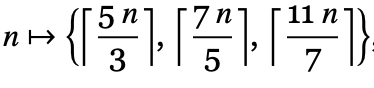, this time experimenting with possible foliations. Nine such foliations are given here (using Nik’s ever-helpful GraphFoliations function):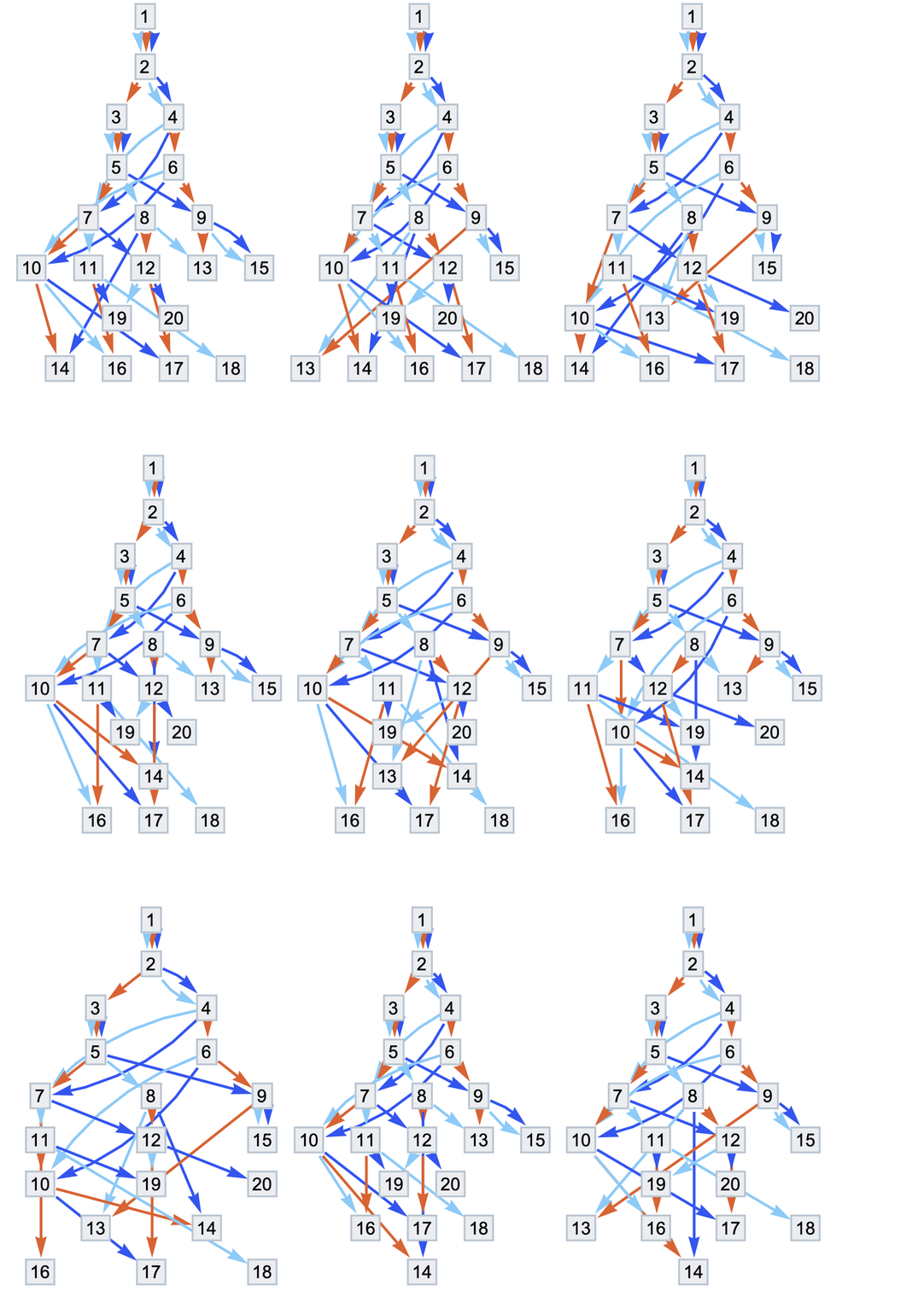✕

Each foliation includes 8–9 foliation slices (which, in the original Physics Project metamodel, are hypersurfaces). For the first foliation shown, the final slice includes vertices 14, 16, 17 and 18, whereas the final slice in the last foliation contains only vertex 14. In order to better understand the difference between these foliations, we can plot the “cardinality” of each foliation slice (i.e. the number of vertices in each) for 500 foliations of the same multicomputation: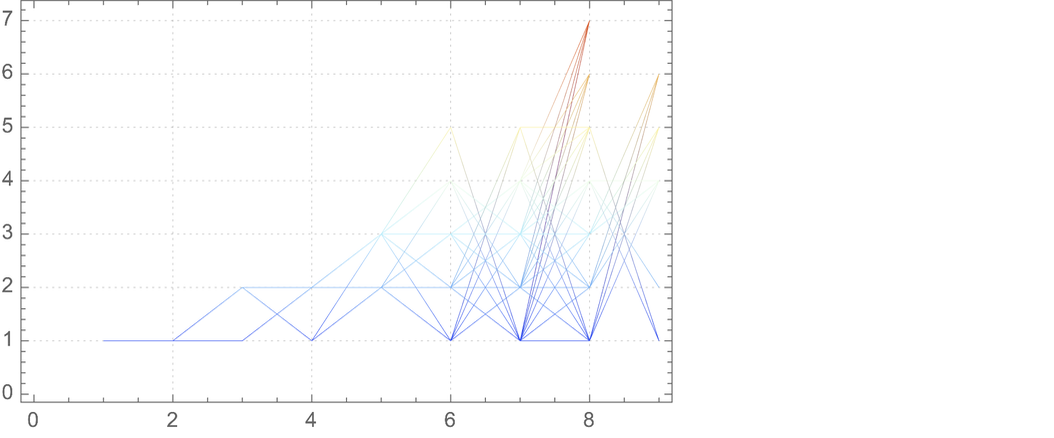✕

We might notice that, even though we take 500 different foliations, we don’t see in the previous plot what appear to be 500 distinct “trajectories.” But we should be able to partially disaggregate the trajectories because there are many foliation slices that share a common cardinality value but include different states. As a proxy measure, we can summate the states in each foliation slice (because, if the numbers differ, then their sums often will too):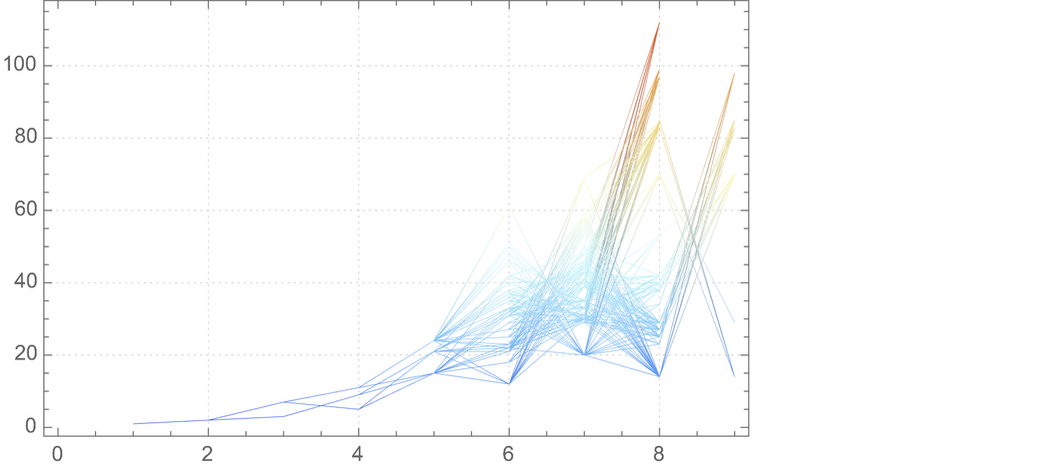✕

Given here is a histogram showing the foliation slice cardinality variance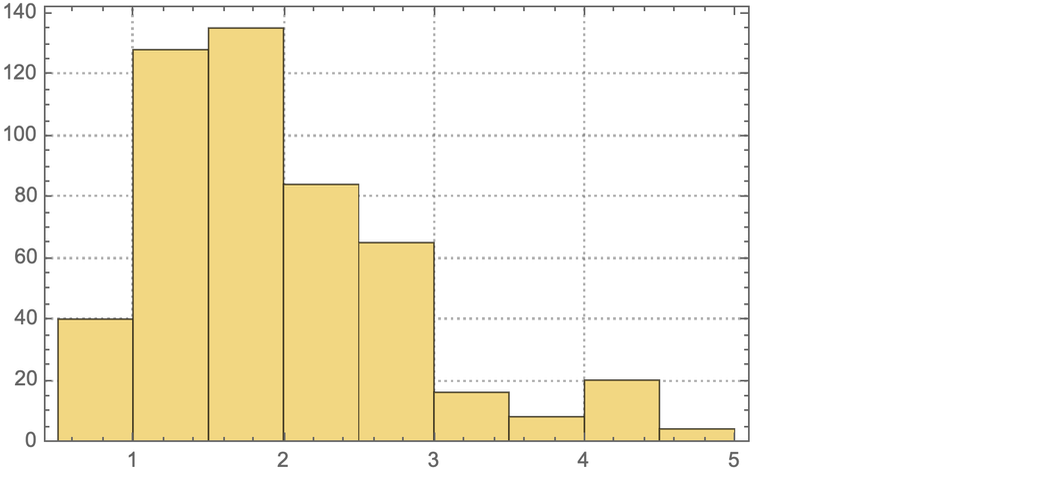✕

and the kurtosis: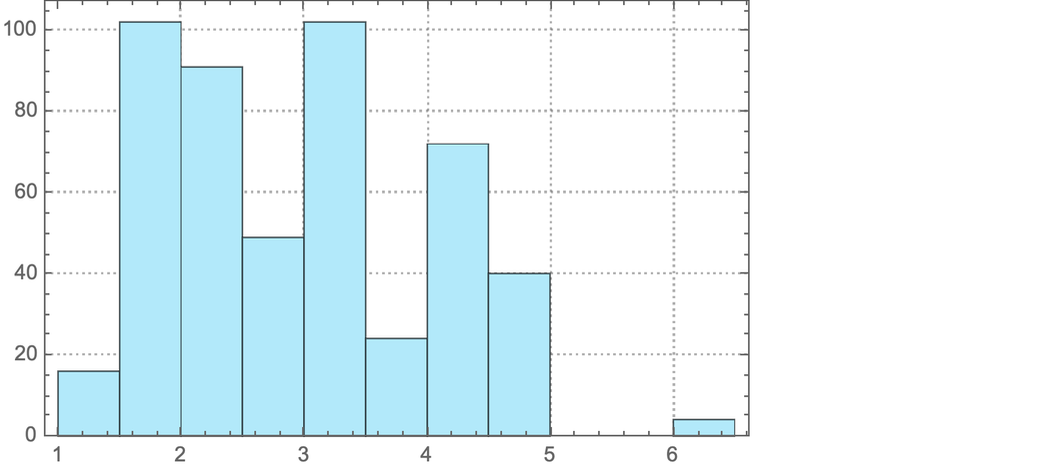✕

It appears as though the most “probable” foliation choices do not minimize cardinality. Thus, foliations are not “all the same,” and if one wants slices that minimize volatility (i.e. variance) or surprisal (i.e. kurtosis), then one must select an “improbable” foliation, which requires careful choice.

As we shall see, careful choice of foliation can mitigate multicomputational irreducibility by allowing one to “observe” the multicomputational evolution without “taking in too much at once.” Let’s consider two random foliations for the rule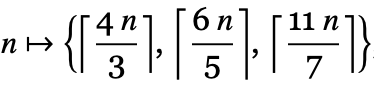, run for seven steps: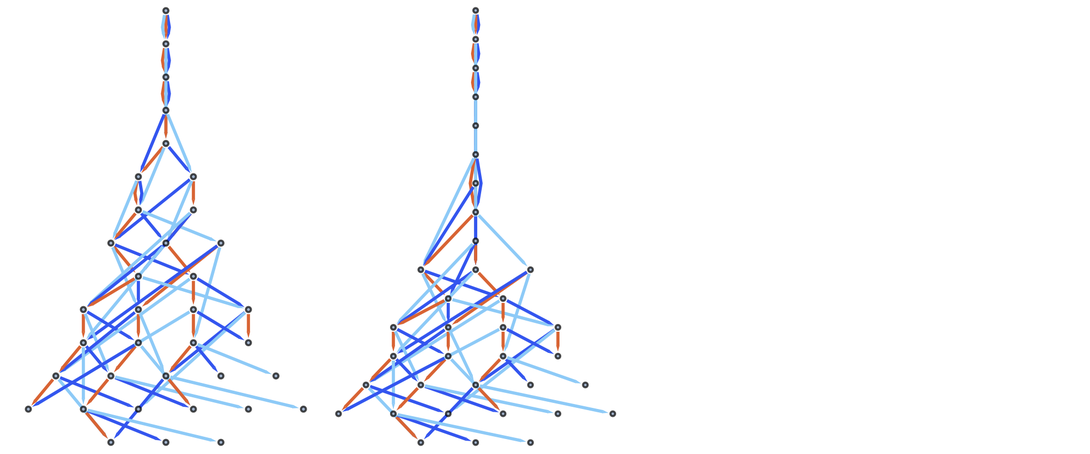✕

And here are array plots for the graph distance matrices for the respective branchial graphs (computed up to 11 steps):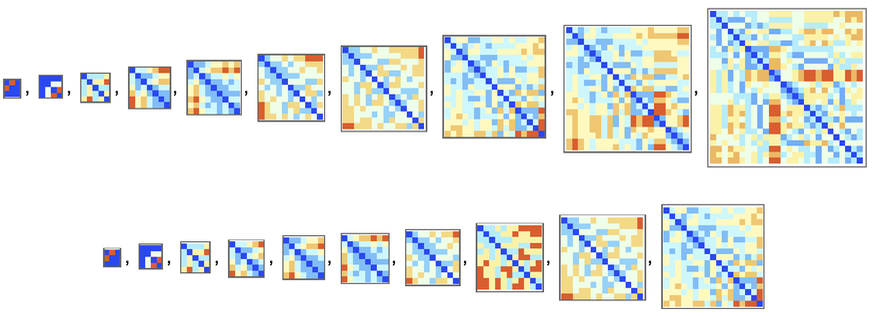✕

Let’s compare their respective λBi values: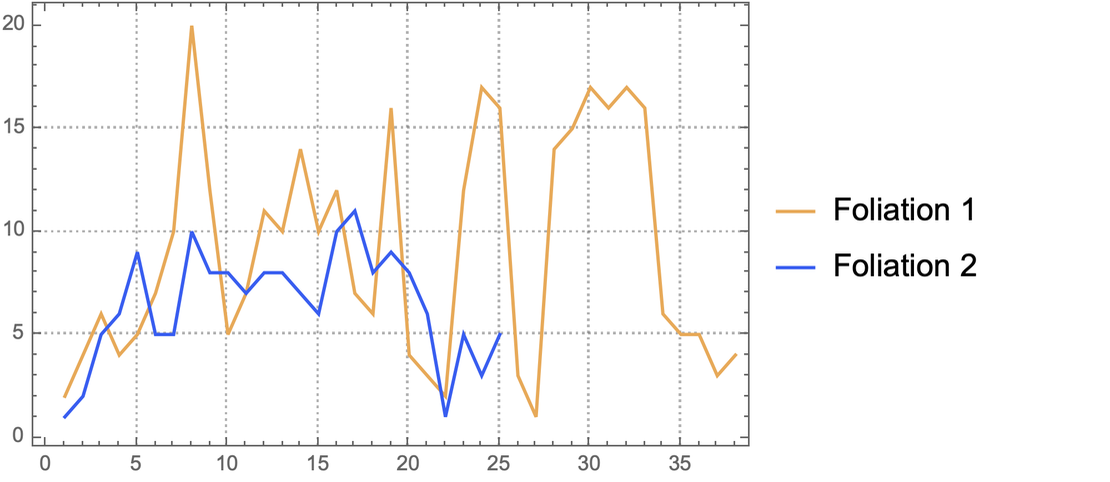✕

Overall, foliation 2 exhibits “tamer” branchial Lyapunov exponent values. And looking at the previous array plots, we see that the evolution of the multicomputation with foliation 2 is more “gradual” than that of foliation 1.

## An Interlude: On Non-Archimedean Reductions and Rulial Primes

Understanding branchial reductions might require honing some unfamiliar modeling sensibilities. This is because branchial distance is non-Archimedean, unlike most graph distances. In a conventional graph, we straightforwardly measure the distance between vertices in terms of the edges that join them. Such a measure of graph distance obeys the Archimedean property in that if the distance d from vertex c to vertex a is greater than the distance d from vertex b to vertex a, it is still the case, for some n, that n×d (b, a) > d (c, a). Put differently, one can multiply n by the distance from b to a and obtain a distance greater than that from c to vertex a. Consider the distance function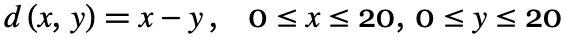computed as a density plot: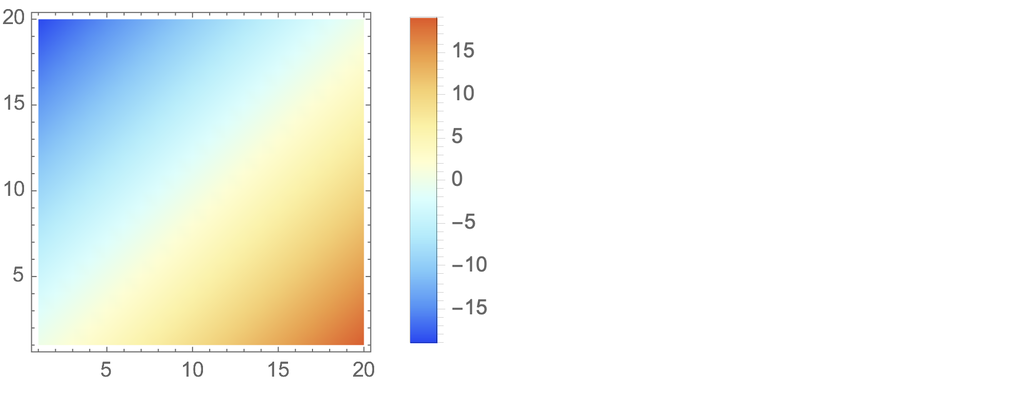✕

Here, d is Archimedean. But branchial distance measures something different. It measures the shared graph ancestry of vertices that may not be joined by edges in the original graph at all; thus, it is non-Archimedean. A common non-Archimedean system in mathematics is that of the p-adic numbers. The p-adic distance dp(x, y) between x and y, for some prime p, is p exponentiated by the reciprocal of the greatest power of p that divides the absolute value of the difference between x and y: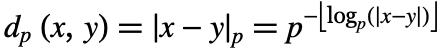Being non-Archimedean, p-adic distance can be difficult to visualize. And p-adic space itself is totally disconnected. But nonetheless, for expository purposes, we can construct a “Euclidean-interpolated” contour space of p-adic distances for –10 ≤ x ≤ 10, –10 ≤ y 10, p = 2, 3, 5, 7, 11, 13. Here, we plot the contours over different surfaces in order to provide a cursory glimpse of the four-dimensional contours: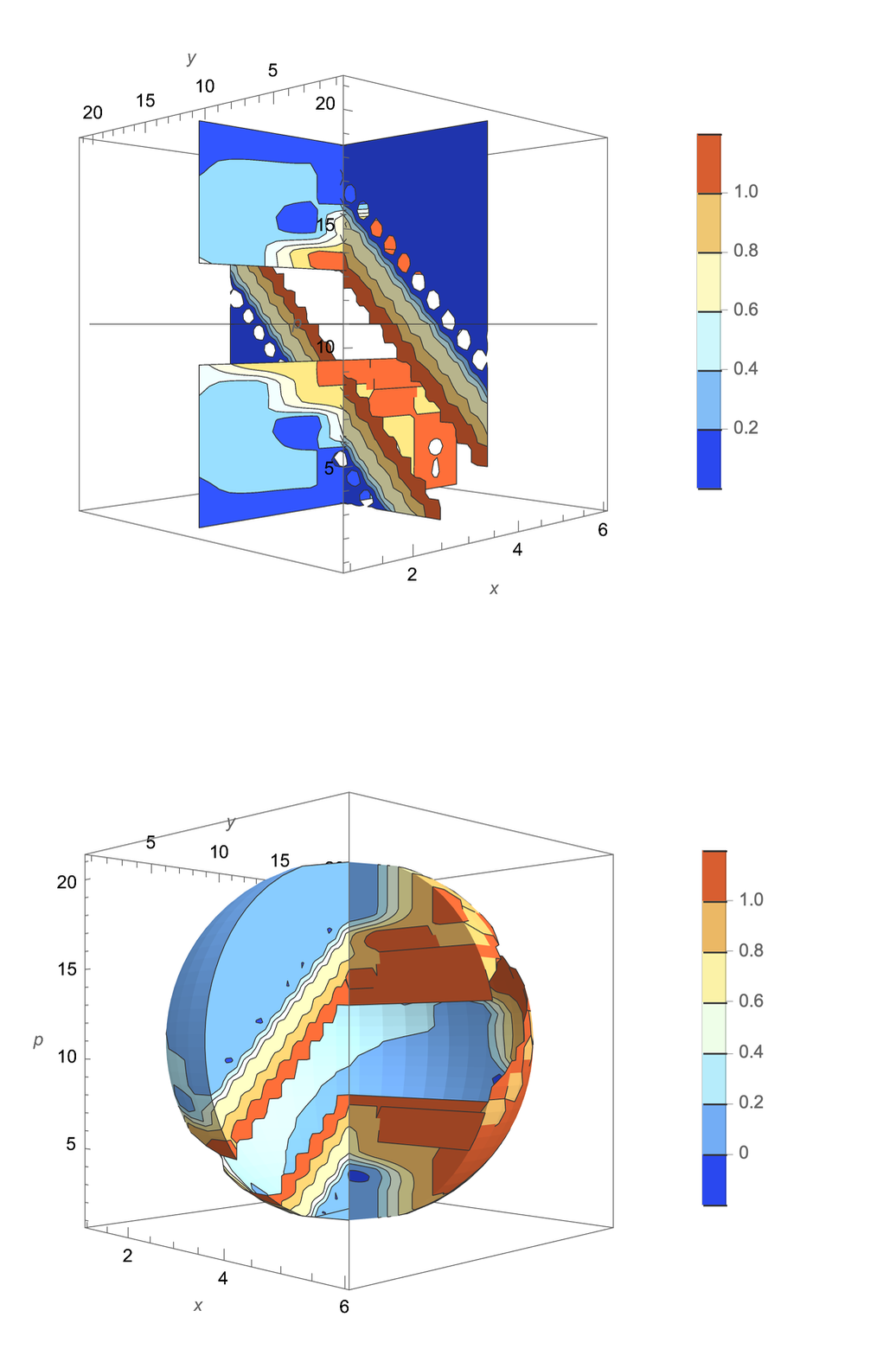✕

Branchial distance and p-adic distance share some similarities. When we measure branchial distance, we are effectively asking, “For any two vertices in a graph, how many steps backward, going from vertices to the prior vertices that feed into those vertices, must we take until we find a common vertex?” Similarly, p-adic distance concerns relationships between numbers in a way that corresponds to prime factorization. In neither case is one asking, “How far in some direction must one travel directly to reach there from here?” Rather, in both cases, one is asking, “How far removed are these two things from one another in terms of common feeders?”

However, the affinities between branchial and p-adic distance become somewhat more concrete when the feeders themselves embody some quality of primality, and primality as such need not be limited to the prime numbers. For instance, consider the case of metamathematical space. One important finding that Stephen made in A New Kind of Science is that, when one lists all possible theorems that can be proved from the axioms of Boolean algebra, it so happens that those that are “named” and are subject to human study are precisely those theorems that “cannot be derived from preceding theorems.” In the following, I plot graphically the dependencies between 60 theorems of Boolean algebra (the same that Stephen considers in the section on empirical metamathematics in the metamathematics piece):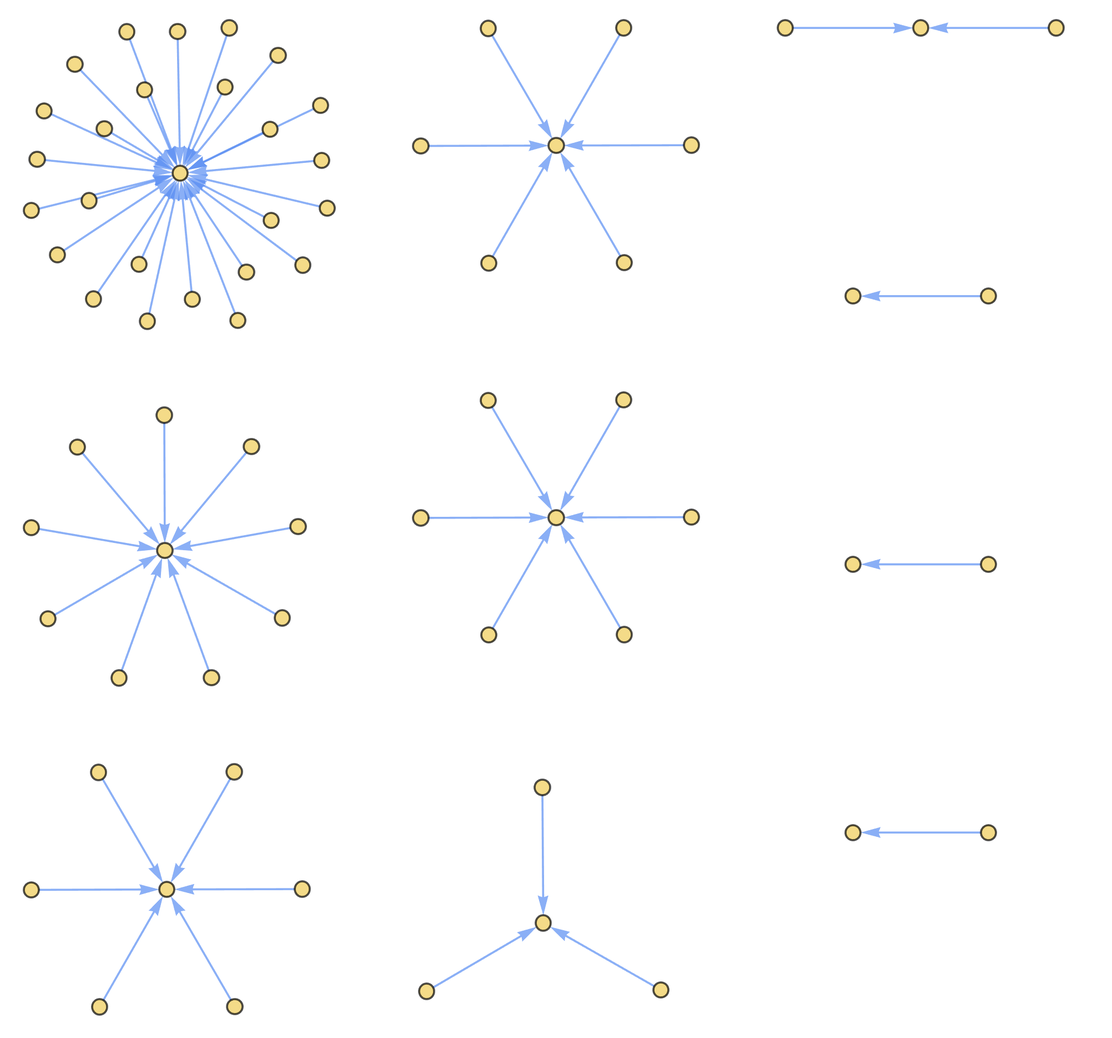✕

Here, each of the named theorems is an attractor, a common ancestor without its own respective ancestor. I propose that ancestorless theorems in metamathematics are a particular case of rulial primes, objects in entailment fabrics (coarsened slices of the Ruliad that serve as reference frames for observers) that are not constructed from the application of a rule to another object. Of course, all objects are constructed from lower-level computations, with the ultimate primordia of the Ruliad being emes. But the point is that multicomputation gives rise to aggregate properties that can be captured by observers (or instruments that transduce values from systems), by merit of interactions between the different evaluation fronts in the multicomputation. And it is in the aggregate context that rulial primes, untethered from lower-level computational dynamics, arise. In the case of Boolean algebra, the theorems that share a rulial prime as a common “sink” are connected in branchial space, such that the branchial distance between each is a non-Archimedean distance taken with respect to a rulial prime (which is not too different, conceptually, from p-adic distance).

Thus, a branchial 2-machine reduction might not simply be an expedient gadget; it might be important in its own right. It might help us to identify particular bulk objects that “stand out” in systems.

## ϱ-Varieties: A Multicomputational Response to Arithmetic and Algebraic Geometry

Multicomputation is a paradigmatic successor to computation, with computation itself already being a successor to the mathematical paradigm. However, it is perfectly possible for multicomputation to “reach backward” two paradigms and metamodel mathematics, even in exotic ways. But multicomputation should also allow us to compute certain objects of mathematics that are not in the canon of the mathematical paradigm, precisely because they involve multicomputational irreducibility and thus cannot be easily studied without some experimental, generative procedure.

For a numerical multicomputation, confluence occurs when different rules yield the same value; that is, their outputs agree. And these outputs are just values that satisfy multiple rules. But the idea that we can study values that satisfy multiple formal specifications is not new. In areas of mathematics such as algebraic geometry, we study common values that satisfy systems of polynomial equations (known as solutions or “roots”) as spaces, known as algebraic varieties. A well-known example of an algebraic variety is an elliptic curve, consisting of solutions to the equation y2 = x3 + a x + b. The following are examples of this particular algebraic variety with values for a and b toggled: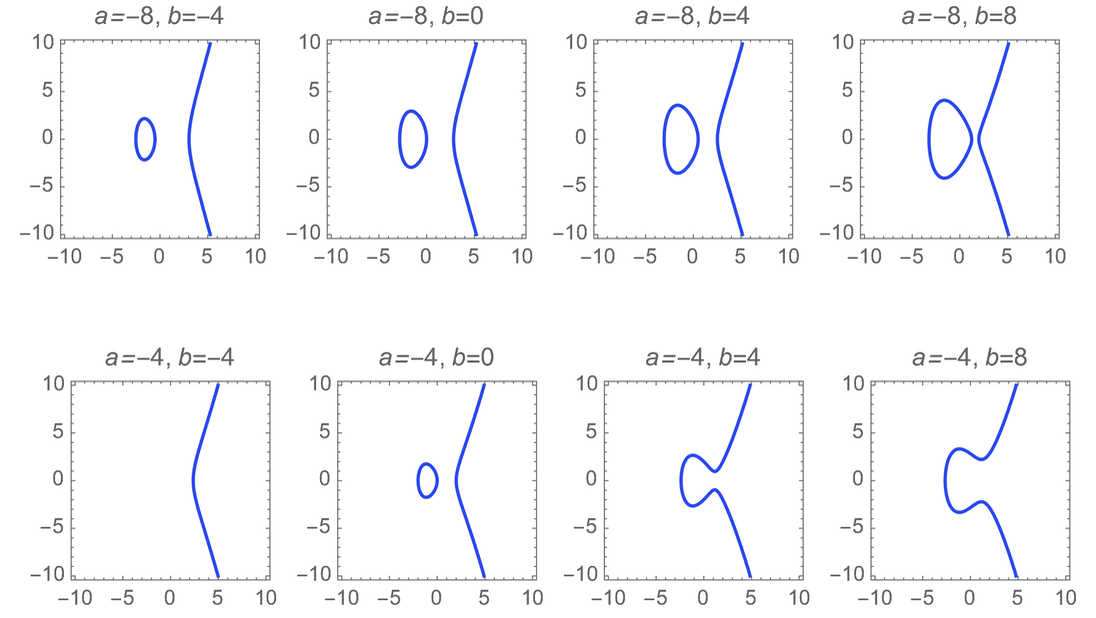✕

And in arithmetic geometry, one seeks to answer questions such as the number of solutions admitted by a variety. Arithmetic geometry is sometimes described as the study of the “complexity” of varieties, though perhaps it doesn’t capture as much complexity as it could.

In the case of multicomputation, however, we don’t study static equations. We study systems composed of rules, such as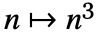, which can be iterated for an indefinite number of steps. Were we to write such rules as equations, we would use recursive functions; in this case,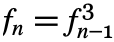, where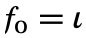for some initial condition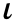. And, for many multicomputations, one can extract from the overall multicomputation the graph of its confluent values, which I will call a rulial variety (shortened as ϱ-variety).

For something generative like a ϱ-variety, it is much more interesting to study the behavior with which confluent values appear than the total number of “solutions” (which, in arithmetic geometry, we would approximate using some height function). And this behavior can be multicomputationally irreducible.

Consider the multicomputation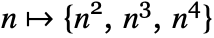. In the following, we begin with initial condition 2 and multicompute for two steps: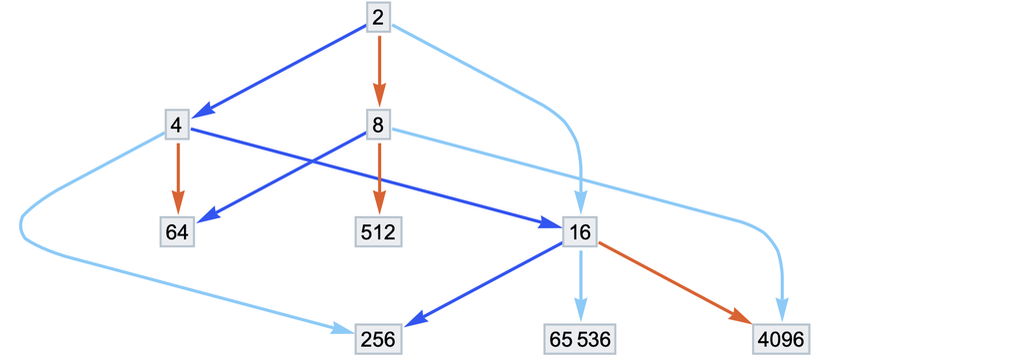✕

And here, we multicompute for eight steps: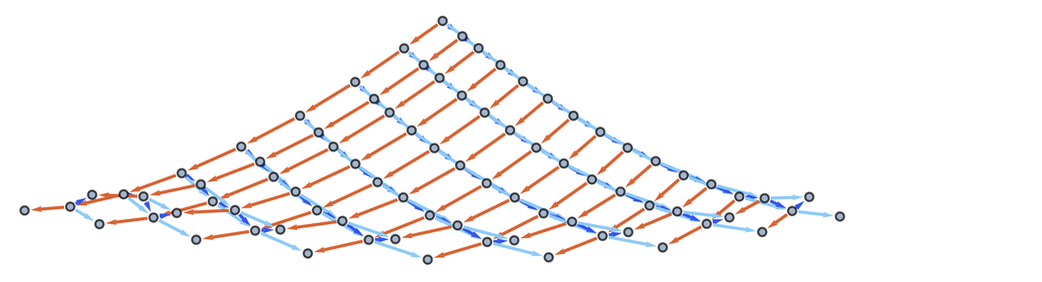✕

There are vertices in these graphs with indegree three (maximal indegree); that is, there are values that satisfy all three rules. Thus we can, in turn, extract from this eight-step multicomputation a ϱ-variety, which is a “subgraph” composed of all vertices with maximal indegree. Indegree must be maximal, for vertices with less than maximal indegree are not numerical values that satisfy all rules. The ϱ-variety for the eight-step multicomputation is shown here: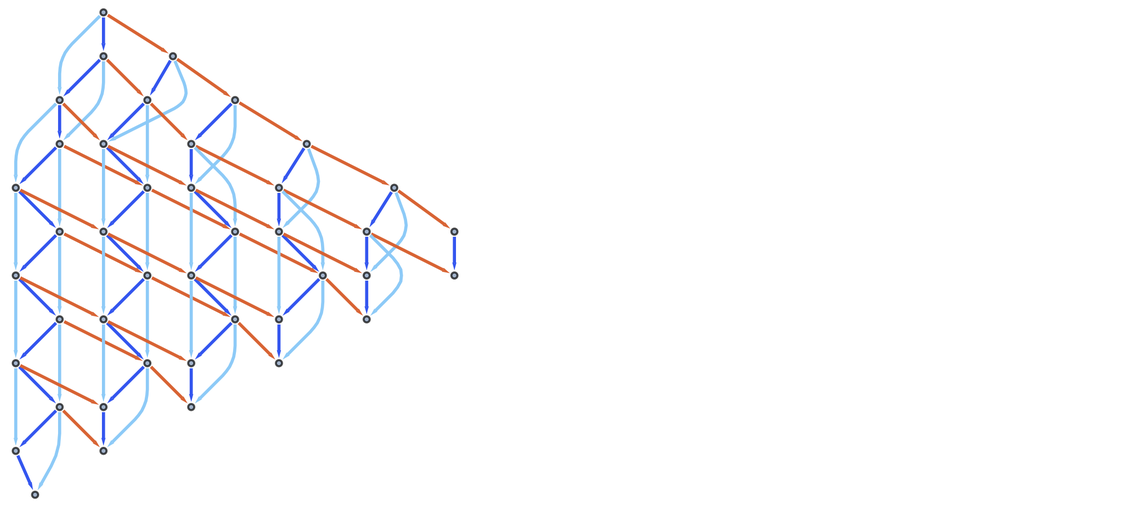✕

This ϱ-variety possesses notable properties. For instance, it appears to be the case that, if we compute the eight-step ϱ-variety for the rulewith any two initial conditions, the resulting ϱ-varieties are isomorphic to one another. Here are the eight-step ϱ-varieties for initial conditions four and five: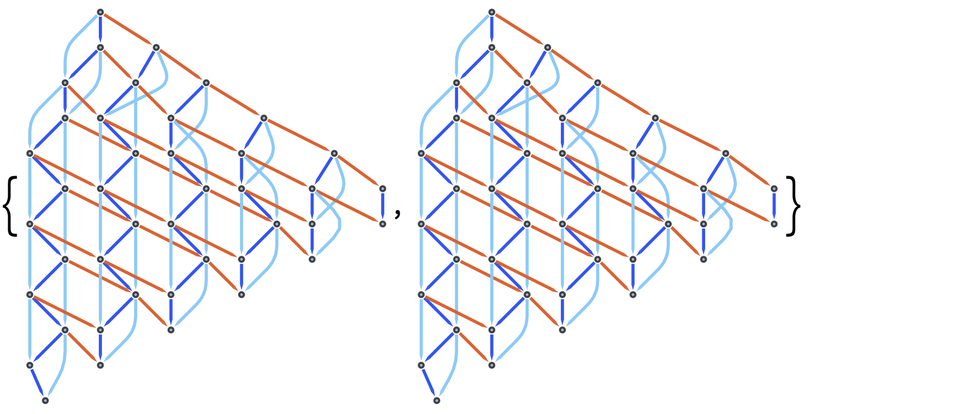✕

They look isomorphic. And indeed, we can prove such to be the case: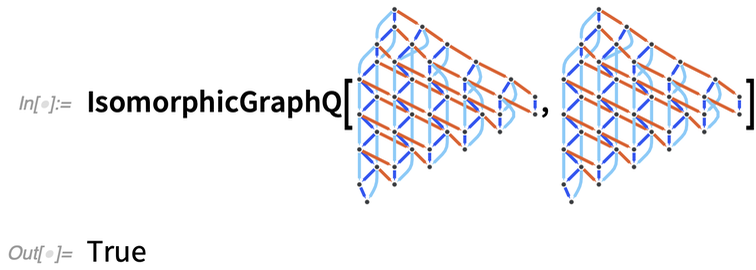✕

And this suggests that, for the rule, one could take the ϱ-variety for all initial conditions and obtain a holochaotic moduli space composed of initial conditions in an isomorphism class (with “holochaotic” being a portmanteau of χάος and λος to connote “possessing all initial states”). And, in principle, one can generate multicomputations that begin with different initial conditions, which makes it easy to study chaos.

We can also continue to yield sub-ϱ-varieties, or subgraphs of ϱ-varieties that are in turn their own respective ϱ-varieties. And we do so by forming a subgraph of vertices that themselves have maximal indegree in the ϱ-variety itself. For the multicomputation, we can yield two further sub-ϱ-varieties, the last of which is a minimal sub-ϱ-variety: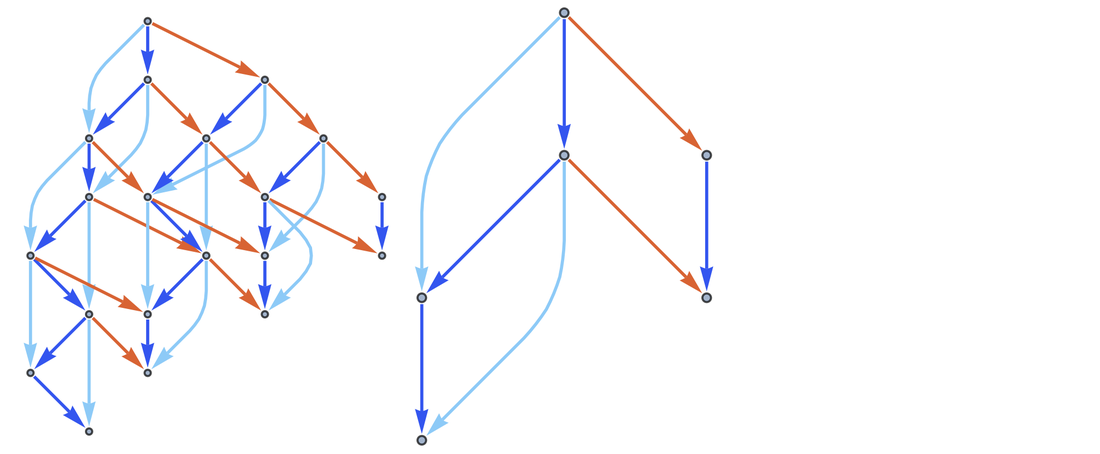✕

Finally, consider the original ϱ-variety and its sub-ϱ-varieties together: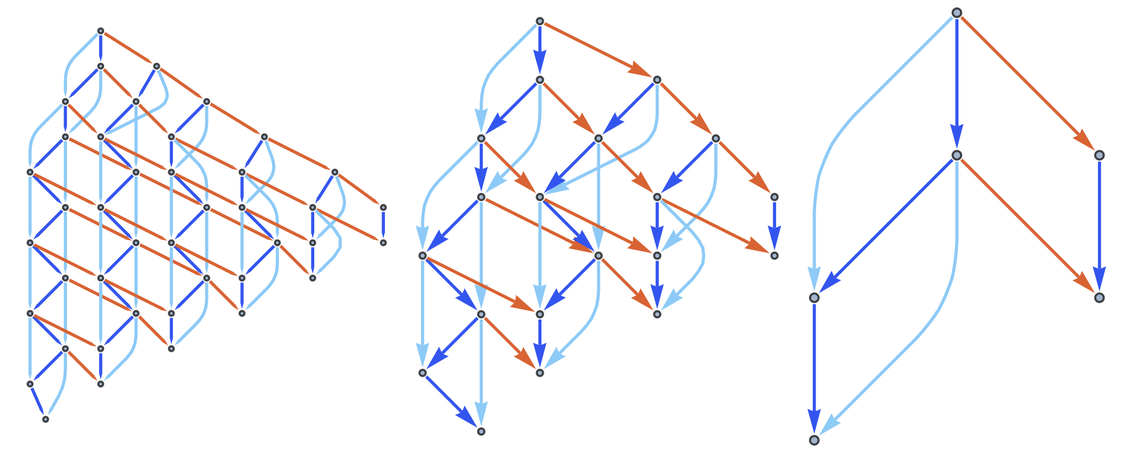✕

As one can see, the branchial graphs for the ϱ-variety and the first sub-ϱ-variety consist of paths that are all interdeformable (with the minimal sub-ϱ-variety possessing only one branchial path):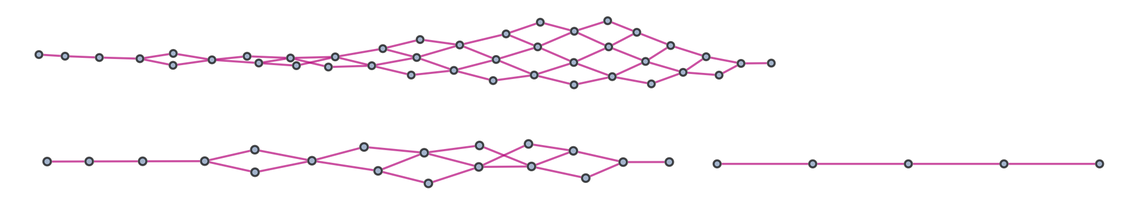✕

Thus, it appears to be the case that the branchial 2-machine reductions are decidable.

Now, let us once again examine the nettlesome multicomputation. Here are the multicomputations for initial conditions 2, 3 and 4: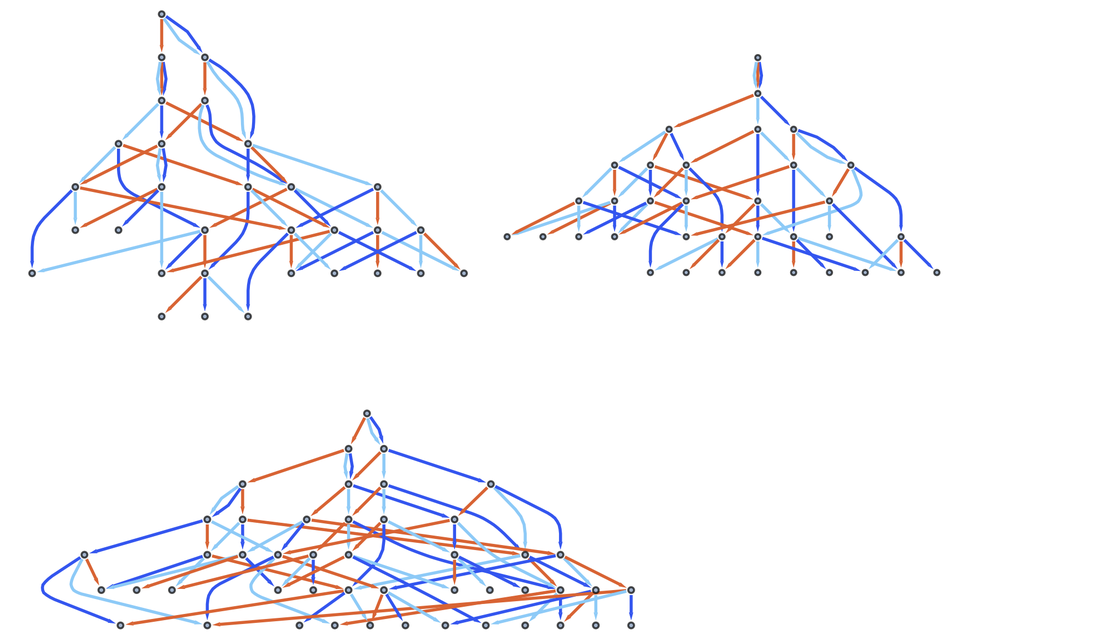✕

Next, we can compute their respective ϱ-varieties: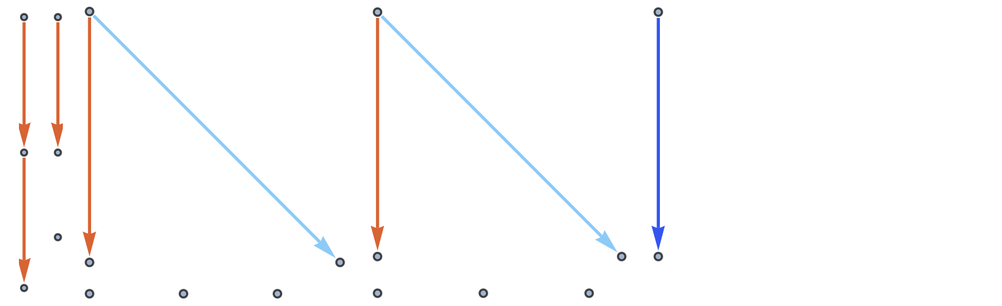✕

These are clearly not isomorphic: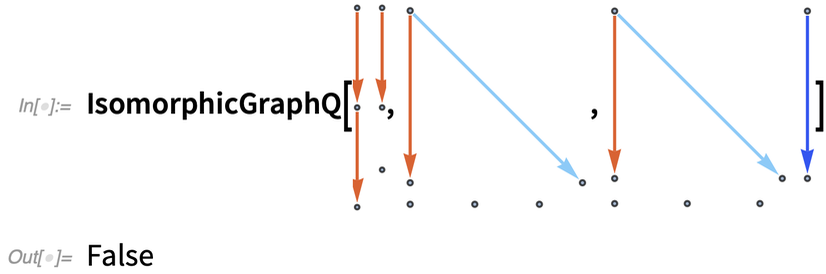✕

And the distance matrices for their respective branchial graphs differ considerably (chaotically) according to choice of initial condition. Here are array plots for graph distance matrices for 15 different initial conditions (ranging from 3 to 45, increasing by increments of three):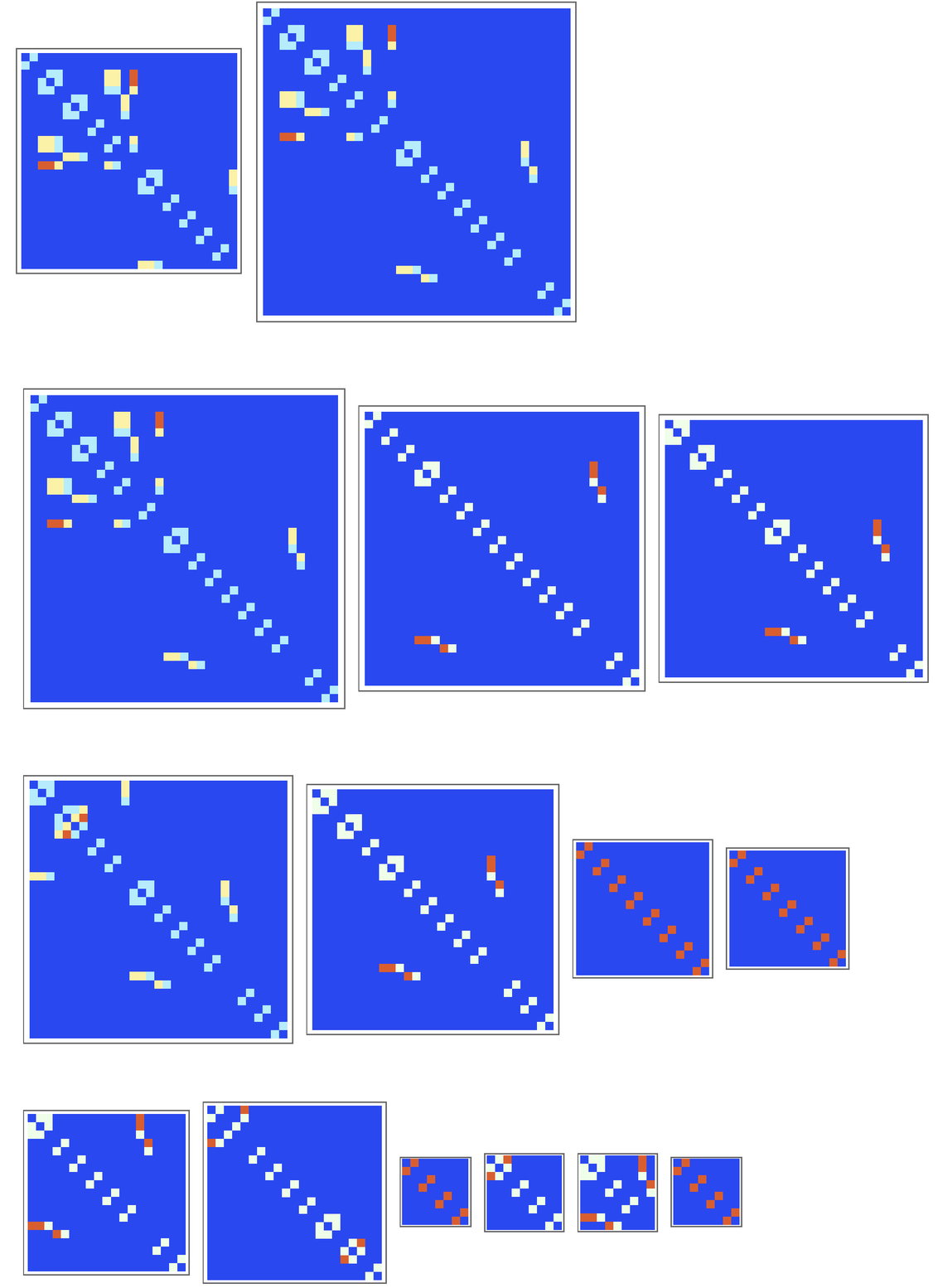✕

We can compare initial conditions by measuring the entropies of the graph distance matrices corresponding to their multicomputations. The lower the entropy, the more uniform the values of the matrix. Here, we plot the entropy over initial conditions 2 ≤≤ 101: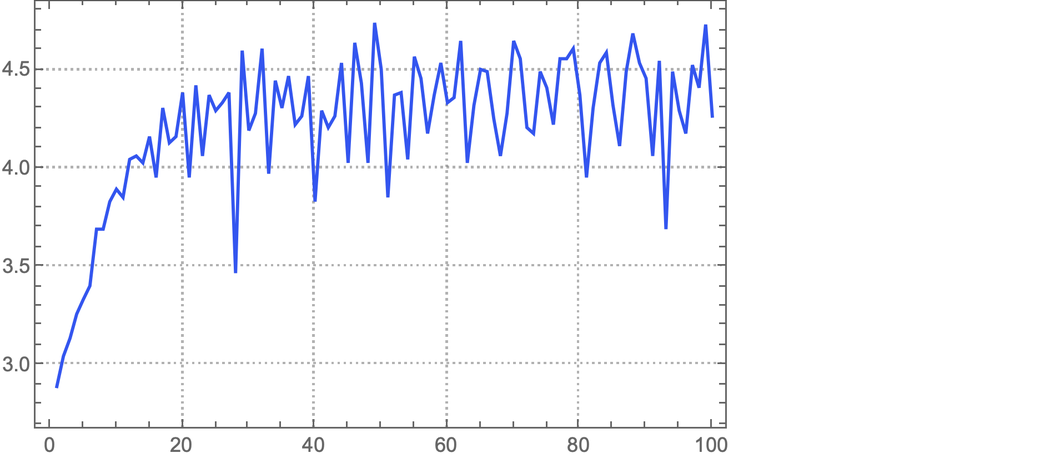✕

Disregarding the cases of small-valued initial conditions, the plot seems to exhibit random walk–like behavior, suggesting that for the rule, one branchial graph for a given initial condition tells us little about the corresponding branchial graph for another. (Note that here we are studying initial conditions for the rule itself, rather than the initial branchial conditions, which we study when we measure λB.)

There is much more to be explored with respect to ϱ-varieties. I introduce them here to reinforce the idea that the behavior of interacting computational rules (such as satisfaction of common values) can be studied behaviorally (i.e. ruliologically) rather than in limit cases (as arithmetic geometry does when estimating the number of solutions for systems of polynomial equations). And, what is more, there are many questions of multicomputational irreducibility (as well as chaotic and holochaotic behavior) that can be examined when studying ϱ-varieties and sub-ϱ-varieties.

## Some Next Steps: Metamodeling Physics

For those interested in straightforward, initial projects on multicomputational irreducibility, the Wolfram Physics Project presents a few opportunities. It is suspected that multicomputational irreducibility is an important concept for metamodeling quantum interference. (In fact, it was during a conversation with Stephen on the topic that I first proposed the concept of multicomputational irreducibility.) There also exists a Registry of Notable Universes on the Physics Project website, in which the graph distance matrices for Wolfram models are already computed. Thus, it should not be too difficult to identify examples that, at least after a certain number of steps, exhibit irreducibility.

## Conclusion

Multicomputation is just beginning. And it appears as though there are countless open questions that we can consider. A careful reader might note that this bulletin introduces several new concepts and raises many open questions, with the findings provided being specific to selected case studies and lacking in generality. This piece is intended to serve as an invitation, with many ideas and provocations introduced with brevity. As the popularity of this paradigm grows, the open questions raised and case studies suggested in early multicomputational works can serve as guideposts for those who are interested and wish to find a way to make helpful contributions.

The computational paradigm of basic science was motivated by the finding that many computations (which are the most fundamental models of discrete processes that follow rules) are unpredictable and autoplectic in their behavior. Multicomputation is an exciting new paradigm because, thanks to the prerogative of choice afforded by foliations, it appears as though what we had previously thought to be physical limits to the understandability of algorithmic behavior can in fact be “negotiated.” As we proceed from ruliologically studying individual processes to systems of interacting processes, we expect to develop a general theory and metamodeling practice with which we can extract bulk, aggregate properties from multicomputations.

Such a goal might sound ambitious, but our quotidian experience as humans suggests overwhelmingly that, despite the dizzying behavior of low-level processes, we, as observers of the world, enjoy a phenomenology with nice “physical UX” and an “interface of macros.” Thus, the foliation can be thought of as the abstract equivalent of a user interface in the pure sciences. And UIs have been incredibly important in that they have made it possible for more people to technologically harness computational power. And in the case of multicomputation, foliations should allow us to scientifically interface between low-level and high-level computational behaviors (including high-level behaviors corresponding to things in the world that we care about), effectively fashioning a bridge from pure computation to the applied sciences. And we will be pursuing many projects in applied multicomputation at the Wolfram Institute for this very reason.

Lastly, multicomputation provides a way to understand the construction and exploration of the Ruliad; it is constructed theoretically by running all possible computations at once, and we explore it by taking foliations over “slices” of the Ruliad and transporting such foliations across rulial space. More will be written about the exploration of the Ruliad—a paramount scientific imperative of our time, also to be pioneered by the Wolfram Institute—on another occasion.

## A Note of Appreciation

I would like to thank Stephen Wolfram for taking an interest in my ideas on multicomputational irreducibility and providing helpful advice on how to communicate my ideas effectively to others; to Nik Murzin for his identification of errors and points in need of greater clarification; to Hatem Elshatlawy for his suggestions regarding descriptions of foundational concepts; and to Xerxes Arsiwalla for encouraging me to think about long-term directions in which I can take the study of multicomputational irreducibility.</>